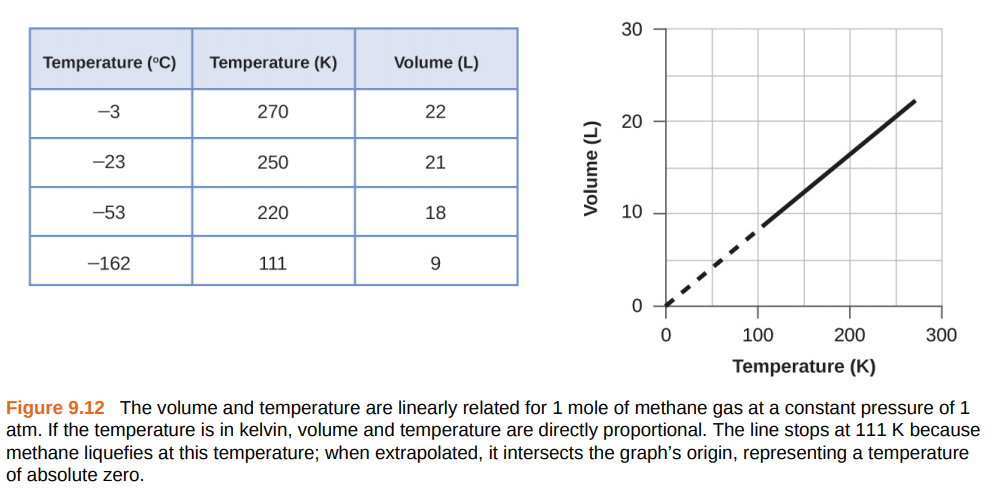Chemistry Practice Problems Chemistry Gas Laws Practice Problems Solution: Determine the volume of 1 mol of CH4 gas at 150 K ...

⚠️Our tutors found the solution shown to be helpful for the problem you're searching for. We don't have the exact solution yet.

# Solution: Determine the volume of 1 mol of CH4 gas at 150 K and 1 atm, using Figure 9.12

###### Problem

Determine the volume of 1 mol of CH4 gas at 150 K and 1 atm, using Figure 9.12Chemistry Gas Laws

Chemistry Gas Laws

#### Q. A high altitude balloon is filled with 1.41 × 104 L of hydrogen at a temperature of 21 °C and a pressure of 745 torr. What is the volume of the balloo...

Solved • Wed Oct 17 2018 11:16:05 GMT-0400 (EDT)

Chemistry Gas Laws

#### Q. A balloon inflated with three breaths of air has a volume of 1.7 L. At the same temperature and pressure, what is the volume of the balloon if five mo...

Solved • Wed Oct 17 2018 11:08:33 GMT-0400 (EDT)

Chemistry Gas Laws

#### Q. What is the temperature of an 11.2-L sample of carbon monoxide, CO, at 744 torr if it occupies 13.3 L at 55 °C and 744 torr?

Solved • Wed Oct 17 2018 11:07:29 GMT-0400 (EDT)

Chemistry Gas Laws

#### Q. A spray can is used until it is empty except for the propellant gas, which has a pressure of 1344 torr at 23 °C. If the can is thrown into a fire (T =...

Solved • Wed Oct 17 2018 11:07:05 GMT-0400 (EDT)